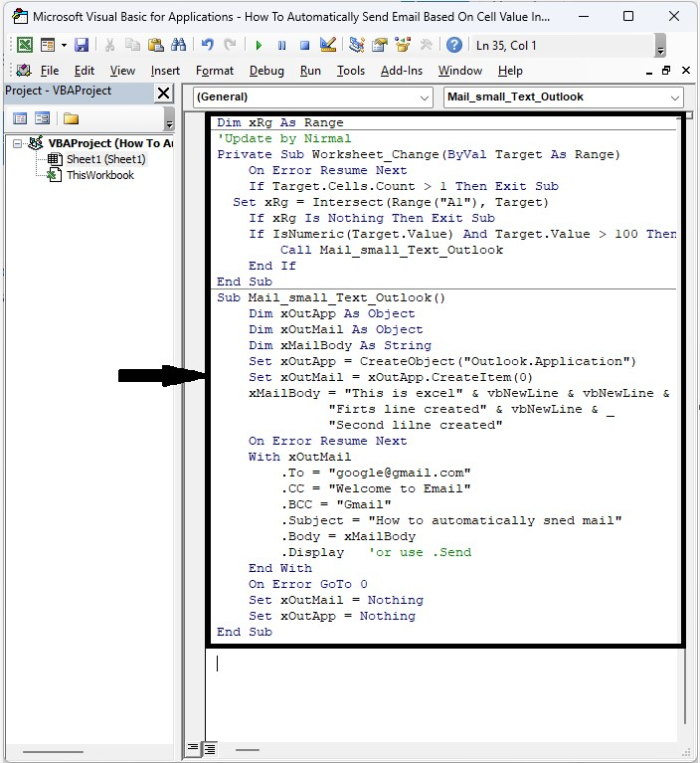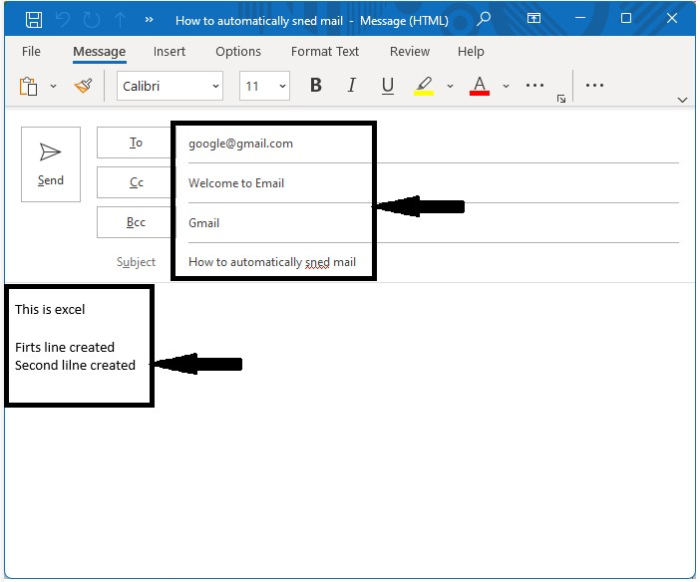# How to Automatically Send Email Based on Cell Value in Excel?

Sending a report from Excel could be a time-consuming and inefficient process if we do it manually. We can automate this process by following the steps below before sending the email. This tutorial will help you understand how we can automatically send email based on cell values in Excel. When the value of the mentioned value follows the condition, then the email application will be opened.

## Automatically Send Email Based on Cell Value

Here we will insert VBA code for the worksheet, then open the email application. Let us see an effortless process to see how we can automatically send email based on cell values in Excel. We will be using the help of a VBA application to complete the process, as it cannot be completed directly in Excel.

Step 1

Consider creating a new excel sheet, then right-clicking on the sheet name and selecting view code to open the vba application, and then typing the programme listed below into the text box shown in the image below.

### Example

Dim xRg As Range
'Update by Nirmal
Private Sub Worksheet_Change(ByVal Target As Range)
On Error Resume Next
If Target.Cells.Count > 1 Then Exit Sub
Set xRg = Intersect(Range("A1"), Target)
If xRg Is Nothing Then Exit Sub
If IsNumeric(Target.Value) And Target.Value > 100 Then
Call Mail_small_Text_Outlook
End If
End Sub
Sub Mail_small_Text_Outlook()
Dim xOutApp As Object
Dim xOutMail As Object
Dim xMailBody As String
Set xOutApp = CreateObject("Outlook.Application")
Set xOutMail = xOutApp.CreateItem(0)
xMailBody = "This is excel" & vbNewLine & vbNewLine & _
"Firts line created" & vbNewLine & _
"Second lilne created"
On Error Resume Next
With xOutMail
.CC = "Welcome to Email"
.BCC = "Gmail"
.Subject = "How to automatically sned mail"
.Body = xMailBody
.Display   'or use .Send
End With
On Error GoTo 0
Set xOutMail = Nothing
Set xOutApp = Nothing
End Sub


In the code, the A1 is the cell where we enter the value >100, which is the condition to open the Outlook application, and we can change the email, CC, BCC, subject, and body in the code as we want.Step 2

Now save the sheet as a macro-enabled workbook, close the vba application using the command "Alt + Q", and every time the value in cell A1 is greater than 100, the Outlook application will be opened.Note − This code will only work if Outlook is your mail program; otherwise, an error will occur.

## Conclusion

In this tutorial, we used a simple example to show how you can use Excel to automatically send email based on cell value.